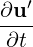### 11.4 Session file configuration: Linear stability analysis

Stability analyses of incompressible flow involves solving the linearised Navier-Stokes equations+ L(U,u′) = -∇p + ν∇2u′,

where L is a linear operator, its adjoint form, or both. The evolution of the linearised Navier-Stokes operator, which evolves a solution from an initial state to a future time t, can be written as

 u(t) = A(t)u(0).

The adjoint evolution operator is denoted as A*. This section details the additional configuration options, in addition to the standard configuration options described earlier, relating to performing this task.

#### 11.4.1 Solver Info

• `Eqtype`: sets the type of equation to solve. For linear stability analysis this must be set to

 Equation Type Dimensions Projections Algorithms UnsteadyNavierStokes 2D, Quasi-3D Continuous VCS,DS
• `EvolutionOperator`: sets the choice of the evolution operator:
• `Nonlinear` (standard non-linear Navier-Stokes equations).
• `Direct` (A – linearised Navier-Stokes equations).
• `Adjoint` (A* – adjoint Navier-Stokes equations).
• `TransientGrowth` (A*A – transient growth evolution operator).
• `Driver`: specifies the type of problem to be solved:
• `Standard` (normal time integration of the equations)
• `ModifiedArnoldi` (computations of the leading eigenvalues and eigenmodes using modified Arnoldi method)
• `Arpack` (computations of eigenvalues/eigenmodes using Implicitly Restarted Arnoldi Method (ARPACK) ).
• `ArpackProblemType`: types of eigenvalues to be computed (for Driver Arpack only)
• `LargestMag` (eigenvalues with largest magnitude).
• `SmallestMag` (eigenvalues with smallest magnitude).
• `LargestReal` (eigenvalues with largest real part).
• `SmallestReal` (eigenvalues with smallest real part).
• `LargestImag` (eigenvalues with largest imaginary part).
• `SmallestIma` (eigenvalues with smallest imaginary part ).
• `Homogeneous`: specifies the Fourier expansion in a third direction (optional)
• `1D` (Fourier spectral method in z-direction).
• `ModeType`: this specifies the type of the quasi-3D problem to be solved.
• `MultipleMode` (stability analysis with multiple modes, `HomModesZ` sets number of modes).
• `SingleMode` (BiGlobal Stability Analysis: full-complex mode. Overrides `HomModesZ` to 1.).
• `HalfMode` (BiGlobal Stability Analysis: half-complex mode u.Re v.Re w.Im p.Re).

Note: For visualization of `Homogeneous` results with `FieldConvert` you can use `--output-points-hom-z` to set output number of modes to a desired value. To process results obtained with `HalfMode` you can convert to `SingleMode` using `FieldConvert` module `halfmodetofourier`.

#### 11.4.2 Parameters

The following parameters can be specified in the `PARAMETERS` section of the session file:

• `kdim`: sets the dimension of the Krylov subspace κ. Can be used with: `ModifiedArnoldi` and `Arpack`. Default value: 16.
• `evtol`: sets the tolerance of the iterative eigenvalue algorithm. Can be used with: `ModifiedArnoldi` and `Arpack`. Default value: 1 × 10-6.
• `nvec`: sets the number of converged eigenvalues sought. Can be used with: `ModifiedArnoldi` and `Arpack`. Default value: 2.
• `nits`: sets the maximum number of Arnoldi iterations to attempt. Can be used with: `ModifiedArnoldi` and `Arpack`. Default value: 500.
• `realShift`: provide a real shift to the direct solver eigenvalue problem by the specified value to improve convergence. Can be used with: `Arpack` only.
• `imagShift`: provide an imaginary shift to the direct solver eigenvalue problem by the specified value to improve convergence. Can be used with: `Arpack` only.
• `LZ`: sets the length in the spanswise direction Lz. Can be used with `Homogeneous` set to `1D`. Default value: 1.
• `HomModesZ`: sets the number of planes in the homogeneous directions. Can be used with `Homogeneous` set to `1D` and `ModeType` set to `MultipleModes`.
• `N_start`: sets the start number of temporal slices for Floquet stability analysis. Default value: 0.
• `N_skip`: sets the number skip of temporal slices for Floquet stability analysis. Default value: 1.
• `N_slices`: sets the number of temporal slices for Floquet stability analysis. Files sequence ```N_start, N_start + N_skip, ..., N_start + N_skip * (N_slices-1)``` will be loaded.
• `BaseFlow_interporder`: sets the interpolation order of temporal slices for Floquet stability analysis. If `BaseFlow_interporder` < 2, the baseflow is taken as periodic and trigonometric functions are used for interpolation. It should be noted that the file `N_start + N_skip * N_slices` is at t = `period` and should not be loaded for the periodic case. If `BaseFlow_interporder` >= 2, the flow is taken as aperiodic and Lagrange interpolation is used. In this case, the file ```N_start + N_skip * (N_slices-1)``` is at t = `period` and should be loaded. Default value: 0.
• `period`: sets the time span (if `BaseFlow_interporder` >= 2) or period (if `BaseFlow_interporder` < 2) of the base flow. Default value: 1.

#### 11.4.3 Functions

When using the direct solver for stability analysis it is necessary to specify a Forcing function “StabilityCoupledLNS” in the form:

1<FORCING>
2   <FORCE TYPE="StabilityCoupledLNS">
3   </FORCE>
4</FORCING>

This is required since we need to tell the solver to use the existing field as a forcing function to the direct matrix inverse as part of the Arnoldi iteration.

Note: Examples of the set up of the direct solver stability analysis (and other incompressible Navier-Stokes solvers) can be found in the regression test directory `NEKTAR/solvers/IncNavierStokesSolver/Tests`. See for example the files `PPF_R15000_ModifiedArnoldi_Shift.tst` and `PPF_R15000_3D.xml` noting that some parameters are specified in the .tst files.